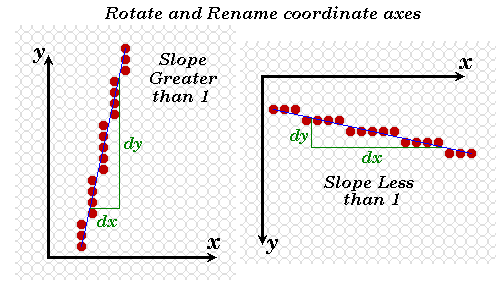# Let's Make it Work!## Solution: symmetry

```public void lineImproved(int x0, int y0, int x1, int y1, Color color) {
int pix = color.getRGB();
int dx = x1 - x0;
int dy = y1 - y0;
raster.setPixel(pix, x0, y0);
if (Math.abs(dx) > Math.abs(dy)) { // slope < 1
float m = (float) dy / (float) dx; // compute slope
float b = y0 - m*x0;
dx = (dx < 0) ? -1 : 1;

while (x0 != x1) {
x0 += dx;
raster.setPixel(pix, x0, Math.round(m*x0 + b));
}
} else	if (dy != 0) { // slope >= 1
float m = (float) dx / (float) dy; // compute slope
float b = x0 - m*y0;
dy = (dy < 0) ? -1 : 1;
while (y0 != y1) {
y0 += dy;
raster.setPixel(pix, Math.round(m*y0 + b), y0);
}
}
}
```

It doesn't make sense to begin optimizing an algorithm before satisfies its design requirements! Code is usually developed under considerable time pressures. If you start optimizing before you have a working prototype you have no fall back position.
Our simple line drawing algorithm does not provide satisfactory results for line slopes greater than 1.

The solution: symmetry.The assigning of one coordinate axis the name x and the other y was an arbitrary choice. Notice that line slopes of greater than one under one assignment result in slopes less than one when the names are reversed. We can modify the lineSimple( ) routine to exploit this symmetry as shown above.

 Web www.vrarchitect.net